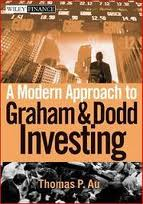Monday 2010-09-20A Modern Approach to Graham and Dodd Investing by Thomas Au

Au needs to work through an introductory physics text to the point where dimensional analysis becomes second nature. When he covers valuation, he makes/repeats several problems regarding money, money over time, and money over time over time; basically the same basic problems that you help fix by always writing your units down: \$, \$/year, \$/(year^2).

```Earnings = \$/year
Value    = \$
Value \$  = Earnings \$/year * Duration years
```

In contrast to the 1920s, the 1930s were a time of economic hardship, a step backward in the unfolding of the American dream. This was probbly the least favorite decade for most people old enough to remember it.
This requirement meant that a qualifying stock's P/E could be no more than 1/2r, where r is the AAA bond rate, measured in decimals.
The company (RJR Nabisco)'s basic problem was tht it had taken on too much leverage in the 1989 leveraged buyout, so aptly recounted in Barbarians at the Gate. So even a modest downturn in sales and profits during the 1990 recession caused a plunge in the value of the bonds. At Buffett's purchase price of around \$60, , the yield to maturity was about 15 percent. But the company was busily selling assets to raise cash, and planned an equity issue that would enabled it to retire the debt. This it did several months after Buffett's purchase, and gave Berkshire a gain of 75 percent (counting interest) during that time span.
Expanding the PEG ratio, which is the P/E ratio divided by the growth rate, some investors and others derived the PEGY ratio, which is the P/E ratio divided by the sum of the (earnings) growth rate G and dividend yield Y. That is, PEGY = (P/E) / (G+Y) with units: (P dollars / E (dollars / year) ) / ( G (no units) + Y (no units)) )
where G has no units = (Earnings next year \$/year - Earnings this year \$/year) / Earnings this year \$/year
and Y has no units = \$ Dividend in one year / Market Value \$
PEGY = years = dollars / (dollars / year)
P/E = years of undiscounted earnings until the company earns "back" your purchase price
1/(G+Y) = present value multiple when discounting by (earning growth rate + dividend rate) in perpetuity
Why do they compare those two? is discounted versus undiscounted meaningful?
Graham's Price, Earnings model:
Price / Share = Earnings / Share (8.5 + 2 (expected earnings growth)) with units: \$ / share = \$ / (year share) ( magic + no units )
\$ / share =? \$ / (year share)
"Poor Mexico, so far from God, so close to the United States."